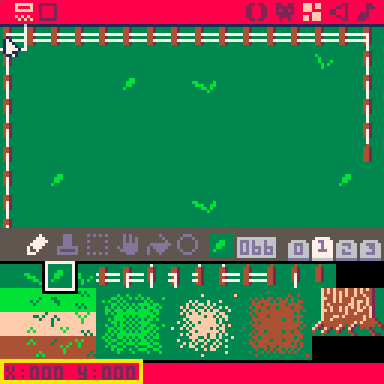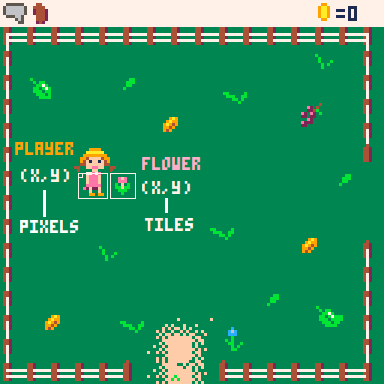# MGET

The `mget()` function in PICO-8 is used to retrieve the sprite number of a tile on the map. It takes in two arguments, column and row, which represent the coordinates of the tile you want to retrieve.

``mget( column, row )``
 mget "map get" column number of tiles from the left (8 pixels in 1 tile) row number of tiles from the top (8 pixels in 1 tile) returns: sprite number

If you open the map editor, and hover your mouse over the map canvas, you can see the column and row number as x and y values in the bottom left corner.#### Get Map-Drawn-Sprite near Code-Drawn-Sprite

Often times, you will be drawing sprites to the screen using pixel coordinates, but this function uses tiles instead of pixels. So to use this function alongside sprites that you draw on your screen using code instead of using the map, you will probably need to convert pixel coordinates over to tile coordinates and that can be as simple as by dividing by 8. Here's an example using a player that is at position (X,Y), and finding the map tile to the right of the player based on the player's pixel coordinates.In this picture, we have the player that is standing at about (30,50) in terms of pixels. And the flower to the right of her is at map position (5,7) in terms of tiles. So here is how we can check what sprite the player is standing next to in code using `mget()`.

``````player_column = player_x / 8
player_row = player_y / 8

sprite = mget( player_column + 1, player_row )``````

First we convert the `player_x` into a map column by dividing by 8. Then we do the same with `player_y` to get the map row. Finally, we create a variable named `sprite` to get the sprite number that will be returned by `mget`, after it checks the correct column and row. Notice that the code adds 1 to the column number so that `mget` will actually look 1 tile to the right of the player and find the flower.

This is useful for finding certian things on the map near the player. For example, you might want to check for a door on the map or walls for collision.#### Checking Sprite Flags on the Map

You will also probably want to use `mget` alongside sprite flags. Sprite flags let you group sprites of a certain type together. So in the above image, you can see a pink flower and a blue flower. If we wanted to check if the player stands next to a flower, then we need to check the sprite numbers of both flowers. But if we used flags instead, then we can make both the blue and pink flowers have the same flag turned on.

Once you have flags turned on to group sprites used on your map, you can use `fget( sprite_number )` to get the flag number. Notice that `fget` takes a sprite number, which is exactly what `mget` returns! So you will often see PICO-8 games that do two things at once:

``flag = fget( mget( tilex,tiley ) )``

These are two functions that are often used together and this might look strange to place one function as an argument of another function, but it's a great way to make your code more efficient. To demonstrate that, here is what that same code would look like if we wrote it out on multiple lines:

```sprite_number = mget(tilex, tiley)
flag = fget(sprite_number)
```

So we are saving the sprite number that mget returns in a variable, then immediately using that variable to pass to fget in order to get the flag. But if that's the only reason we get the sprite number, then it's a waste of setting a variable. However, it is easier to read and understand what the code is doing, so you'll see programmers writing it the second way for that reason.564

10 Apr 2023

`Font`